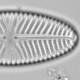## Von Neumann’s elephant’s trunk wiggles at WUWT

With four parameters I can fit an elephant, and with five I can make him wiggle his trunk
Attributed to John von Neumann by Freeman Dyson via Enrico Fermi

Von Neumann’s elephant has found fertile ground at WUWT, where today R. J. Salvador is the mahout commanding the wrinkled pachyderm to wiggle its trunk so prominently that even some the regulars can detect the proboscis.

Salvador finds a correlation between global temperature anomaly 1880-2013 and sunspot numbers processed by a model with five arbitrary parameters that have no obvious physical meaning. Apparently we are supposed to be impressed by an r2 of 0.82. But since the value of the five parameters were chosen to maximise the fit between sunspot number and the temperature anomaly, a strong correlation might be expected.

TA= d*[Σcos(a*SN)-Σb*SNc]+e from month 1 to the present

where TA is the temperature anomaly, SN is the sunspot number and a, b, c, d, e and constants.

Constants d and e are scale parameters and don’t affect the r2 between estimated and measured temperature anomaly (as d is negative (mistakenly labelled e in Salvador’s post) the sign of the correlation is switched).

The component Σb*SNc is more interesting. As SN is always zero or greater (and b is positive), this component monotonically increases, quickly where SN is high, and slowly when it is low. Despite these changes in slope, this component basically just adds a trend to the estimates, and can be adjusted until the trend matches the observed trend. It has no physical meaning.

The component Σcos(a*SN) is more interesting still. This is calculated in radians: 2*pi ≈ 6.28 radians gives one complete cosine cycle. Parameter a is estimated as 148, so a change in the sunspot number of just one gives us 148/6.28 ≈ 23 cosine cycles. Very small changes in SN give large changes in cos(a*SN), so cos(a*SN) is effectively an easy (and very bad) way to generate pseudorandom numbers, with a tendency to have more values near +1 and -1 because of the shape of the cosine wave.cos(a*SN) against SN for SN values between 100 and 101. SN values are reported to one decimal place, these values are highlighted in red.

Summing these pseudorandom numbers will give a random walk. Changing the value of a slightly will give different pseudorandom numbers and so a different random walk. By controlling the single parameter a, a very wide range of shapes can be generated. With enough patience, it should be possible to find a value of a that gives a random walk that resembles global temperature anomalies or any other curve. This component has no physical meaning.Σcos(a*SN) for a=148.4258 (red) and other values of a between 148 and 149 (blue).

Salvador’s calculations are devoid of any physical meaning, they offer absolutely no evidence of a sunspot-temperature relationship despite the impressive r2. They are an elephantine error.

```#import data
tmp<-tmp[1:1598,]
SSN<-SN\$SSN[SN\$YEAR>=1880][1:1598]
mo<-tmp\$year+(tmp\$month-1)/12

a= 148.425811533409
b= 0.00022670169089817989
c= 1.3299372454954419
d= -0.011857962851469542
e= -0.25878555224841393
TA<-sapply(1:length(SSN),function(n)d*(sum(cos(a*SSN[1:n]))-sum(b*SSN[1:n]^c))+e)

plot(mo,tmp\$tmp, type="l", col=2)
lines(mo,TA)

TA2<-sapply(1:length(SSN),function(n)sum(b*SSN[1:n]^c))
plot(mo,TA2, type="l", col=2)

plot(seq(100,101,.001), cos(seq(100,101,.001)*a), type="l", xlab="SN", ylab="cos(a*SN)")
points(seq(100,101,.1), cos(seq(100,101,.1)*a), pch=16, col=2)

x11(4.5,4.5);par(mar=c(3,3,1,1),mgp=c(1.5,.5,0))
TA1<-sapply(1:length(SSN),function(n)sum(cos(a*SSN[1:n])))
plot(mo,TA1, type="l", col=2, ylim=c(-20, 60), xlab="year", ylab=expression(paste(Sigma, "cos(a*SN)")))
TA1s<-sapply(seq(148,149,.2), function(a){
TA1<-sapply(1:length(SSN),function(n)sum(cos(a*SSN[1:n])))
lines(mo,TA1, type="l", col=4)
TA1
})
```Ecologist with interests in quantitative methods and palaeoenvironments
This entry was posted in Fake climate sceptics, Silliness, WUWT. Bookmark the permalink.

### 3 Responses to Von Neumann’s elephant’s trunk wiggles at WUWT

1.Fragmester says:

Saw the original at WUWT and although I wasn’t really following the maths, my science training hit in when the constants were given as up to 20 decimal places. In the world of weather and climate, is anything measured past 3 or 4 dp?

•richard telford says:

There won’t be many variables that can be measured to more than a few significant figures, certainly never 20. However, many people forget to round their numbers off and report as many significant numbers as Excel gives them, which can be many many more than is appropriate. It’s a real give-away that people don’t understand what they are doing. I think that’s the case with R J Salvador. The results are very sensitive to the choice of a between 148 and 149, but with three decimals, 148.425 to 148.426, the difference is hardly noticeable. Yes this is absurd, but not as absurd as needing all 20 decimal places as Salvador implied.

2.WebHubTelescope says:

Nice deconstruction and debunking of one of the craziest and most arbirary models I have seen in a while.